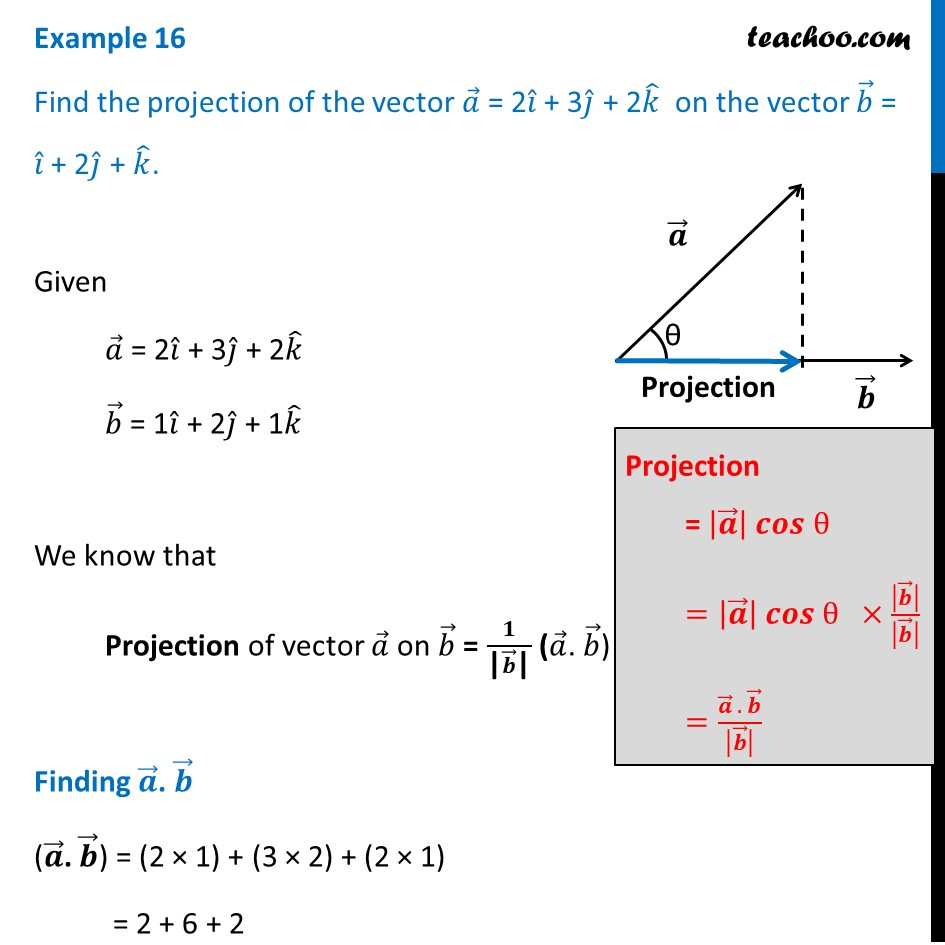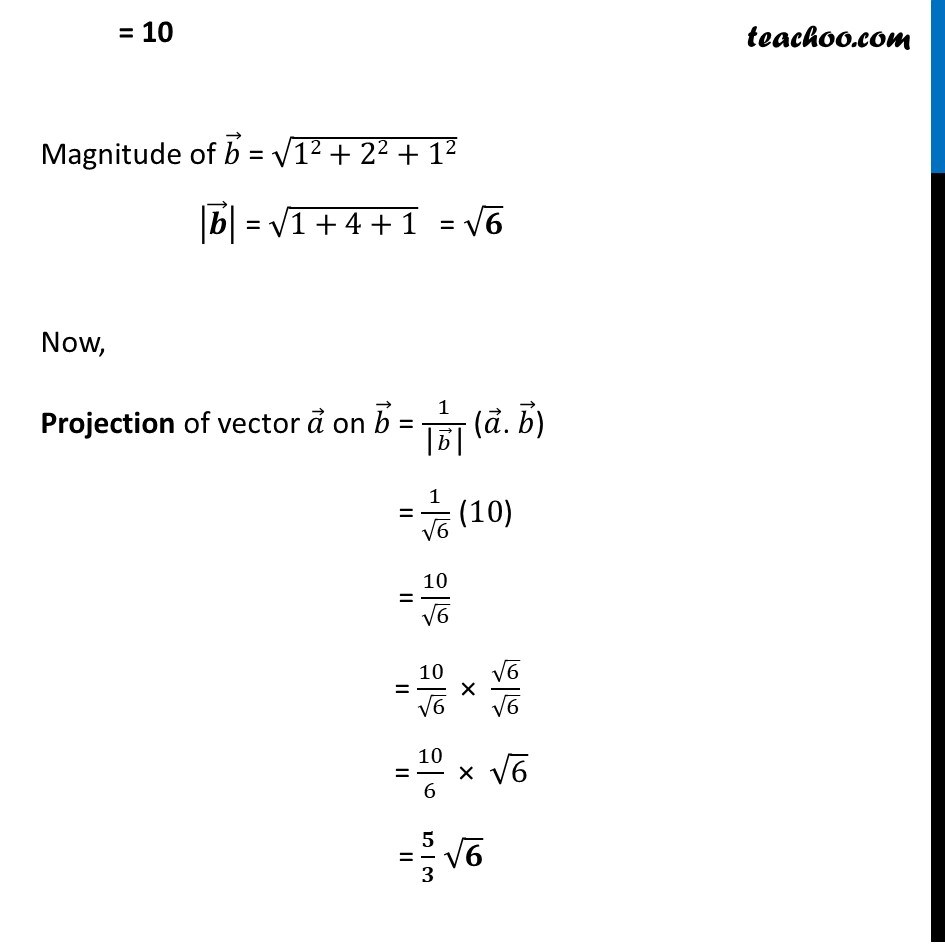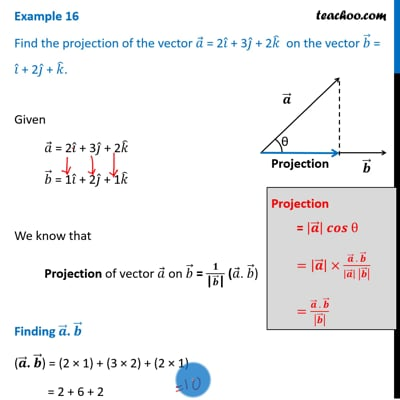Examples

Chapter 10 Class 12 Vector Algebra
Serial order wiseThis video is only available for Teachoo black users

Learn in your speed, with individual attention - Teachoo Maths 1-on-1 Class

### Transcript

Example 16 Find the projection of the vector 𝑎 ⃗ = 2𝑖 ̂ + 3𝑗 ̂ + 2𝑘 ̂ on the vector 𝑏 ⃗ = 𝑖 ̂ + 2𝑗 ̂ + 𝑘 ̂. Given 𝑎 ⃗ = 2𝑖 ̂ + 3𝑗 ̂ + 2𝑘 ̂ 𝑏 ⃗ = 1𝑖 ̂ + 2𝑗 ̂ + 1𝑘 ̂ We know that Projection of vector 𝑎 ⃗ on 𝑏 ⃗ = 𝟏/("|" 𝒃 ⃗"|" ) (𝑎 ⃗. 𝑏 ⃗) Finding 𝒂 ⃗. 𝒃 ⃗ (𝒂 ⃗. 𝒃 ⃗) = (2 × 1) + (3 × 2) + (2 × 1) = 2 + 6 + 2 = 10 Magnitude of 𝑏 ⃗ = √(12+22+12) |𝒃 ⃗ | = √(1+4+1) = √𝟔 Now, Projection of vector 𝑎 ⃗ on 𝑏 ⃗ = 1/("|" 𝑏 ⃗"|" ) (𝑎 ⃗. 𝑏 ⃗) = 1/√6 (10) = 10/√6 = 10/√6 × √6/√6 = 10/6 × √6 = 𝟓/𝟑 √𝟔